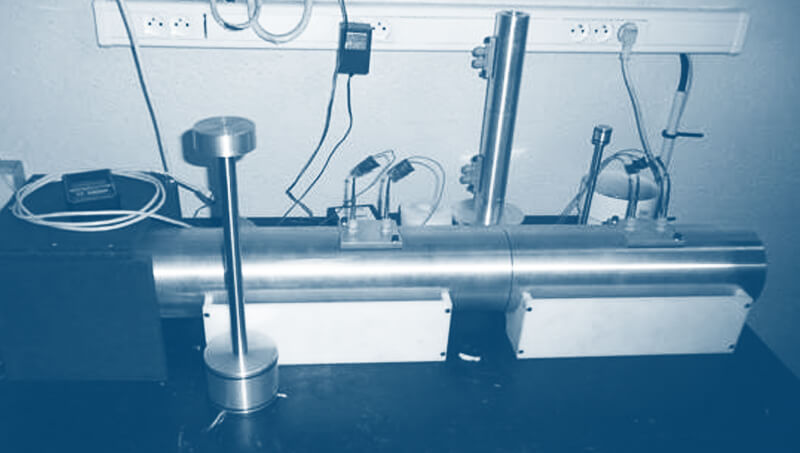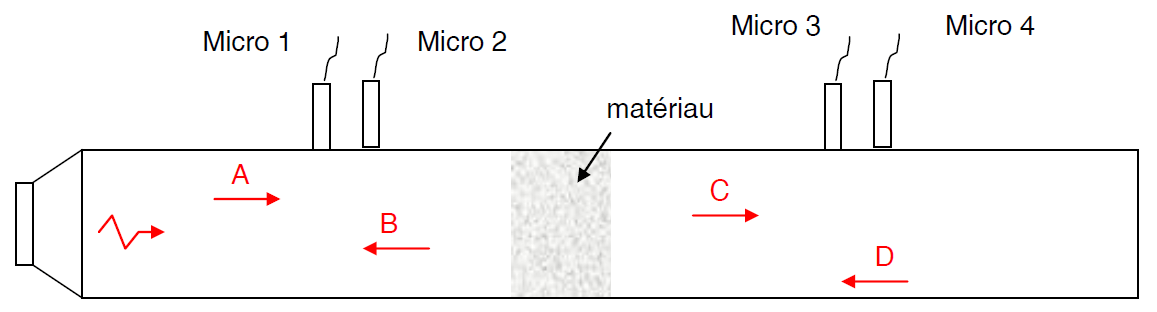Select Page

# Impedance tubeThe CEVAA has two impedance tubes in order to measure the absorbent properties of porous materials.

Our impedance tubes:

• Tube 44 mm (temperature regulated between 0 and 60°C.)
• Tube 29/100mm

Complies with standard NF EN ISO 10534-2.

## Principles of the impedance tube

A loudspeaker emitting a plane wave in a tube in which the sample to be tested is placed as described in the following diagram:Incident wave of complex amplitude A
Wave reflected by the material of complex amplitude B
Transmitted wave of complex amplitude C
Wave reflected by the extremity of complex amplitude D

These waves make it possible to determine the absorption coefficient and the attenuation index.

#### 44 mm tube (temperature regulated)

It makes it possible to determine the acoustic absorption coefficient and the attenuation index between 250 and 4250 Hz. These are measured at normal incidence in the 44 mm impedance tube using the three-microphone method ( and ) complementary to ASTM E2611-09 ().

#### 29/100 mm tube

It makes it possible to determine the acoustic absorption coefficient and the attenuation index between 50 and 6300 Hz. These are measured with normal incidence in the 29/100 mm impedance tube according to the ASTM – E2611-09 standard. .

• Calculation of the absorption coefficient
• Calculation of the attenuation index

#### Test conditions

• Acquisition under LMS software
• Counting under Octave software
Theoretical porous models from experimental measurements can be proposed.

From the measured data, a model of porous material will be identified by inverse method. The acoustic properties of an identified porous model are:

• Open porosity
• Tortuosity
• Resistivity to the passage of air
• Thermal characteristic length
• Viscous characteristic length

These models allow following the study to carry out simulations by the transfer matrix method to predict the performance of multilayers, the performance of a material for an untested thickness, etc. For each material, an abacus of 5 different thicknesses can be made.

## MATERIALS CHARACTERIZATION BENCHES

Poisson's ratio characterization bench

Dynamic mechanical analysis (DMA)